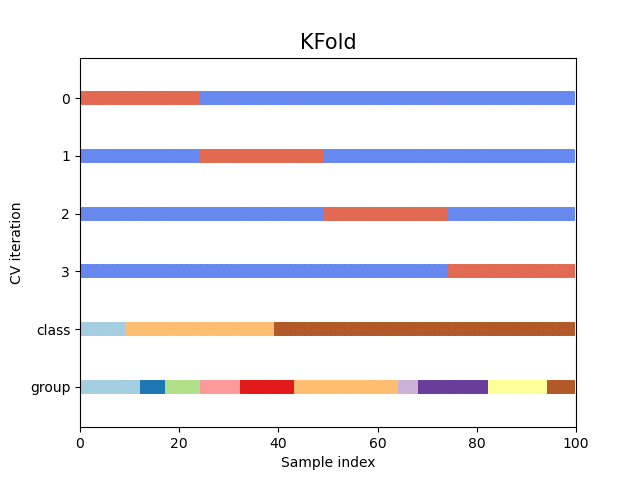# Feature selection¶

## Recursive feature elimination¶

In order to reduce processing time and memory space, we can try to select for important features within the predictors. Again, the sklearn library provides some useful functionality for this:

%%time

from sklearn.model_selection import KFold
from sklearn.feature_selection import RFECV

rfecv = RFECV(estimator=model, step=1, cv=KFold(5), n_jobs = -1, scoring='accuracy', min_features_to_select = 1)
rfecv.fit(df_train[predictors], df_train[[target]])

CPU times: user 5.29 s, sys: 145 ms, total: 5.43 s
Wall time: 12.7 s


The RFECV tool runs a recursive feature elimination on the model. This means, that, starting with all available predictors, the model is iteratively trained and validated with a decreasing number of predictors. After each step, the feature with least importance is removed from the predictor set. The process finally returns the number (and list) of ideally used features.

In our example, we use a simple KFold cross validation with 5 splits on our data at each step. The concept behind a KFold cross validation is described in the sklearn docs and can be visualized like this:The optimal number of features is stored in the n_features_ field:

print("Optimal number of features : %d" % rfecv.n_features_)

Optimal number of features : 9


The model accuracy for each run can be plotted via:

import matplotlib.pyplot as plt

plt.figure()
plt.xlabel("Number of features selected")
plt.ylabel("Cross validation score (accuracy)")
plt.plot(range(1, len(rfecv.cv_results_["mean_test_score"])+1), rfecv.cv_results_["mean_test_score"])
plt.grid(axis="both")
plt.xticks(range(1,len(rfecv.cv_results_["mean_test_score"])+1,1))
plt.show()This shows us, that the model performance slightly increases from 12 to 9 predictors where it reaches its maximum. However, it stay in a high plateau until only ~5 features are left. Afterwards it decreases with a sharp decline.

Features that add value to the model can be listed via:

df_train[predictors].columns[rfecv.ranking_==1]

Index(['B1', 'B2', 'B4', 'B5', 'B6', 'B8A', 'B9', 'B11', 'B12'], dtype='object')


All unimportant features can be listed accordingly:

df_train[predictors].columns[rfecv.ranking_>1]

Index(['B3', 'B7', 'B8'], dtype='object')


As we saw in the plot above, although maximum performance is achieved at 9 features, it might make sense to reduce the feature set to only 5 features to keep the computational load small while reducing the model accuracy only slightly. For this, however, we have to know the importances of the 9 features that are left.

This data is sotred in the field rfecv.estimator_.feature_importances_ (which is not documented in the official docs yet):

rfecv.estimator_.feature_importances_

array([0.57190884, 0.04304589, 0.07288276, 0.01727214, 0.00405035,
0.04302842, 0.20923332, 0.00981012, 0.02876817])


We can plot the importances like this:

feat_imps = pd.DataFrame({"importances" : rfecv.estimator_.feature_importances_, "features": df_train[predictors].columns[rfecv.ranking_==1]})
feat_imps = feat_imps.sort_values(by="importances")

plt.figure(figsize=(10, 6))
plt.barh(y=feat_imps.features, width=feat_imps.importances, color='#1976D2')
plt.title('RFECV - Feature Importances', fontsize=20, fontweight='bold', pad=20)
plt.show()The 5 most important features (which we could reduce the feature set to) would thus be:

• B1

• B9

• B4

• B2

• B8a

## Forward feature selection¶

Another approach to reduce the number of predictors is to use a forward feature selection. This can be realized with sklearn’s SequentialFeatureSelector:

from sklearn.feature_selection import SequentialFeatureSelector

seqFeatSel = SequentialFeatureSelector(model, n_features_to_select=9, direction='forward', scoring="accuracy", cv=5, n_jobs=-1)


As you see, here we have to provide the number of features we want to reduce the predictor set to. Unfortunately, the SequentialFeatureSelector does not provide the optimal number of features to use. So we just provide it with the optimal count that we acquired during the RFE approach from above.

seqFeatSel.fit(df_train[predictors], df_train[[target]])

SequentialFeatureSelector(estimator=DecisionTreeClassifier(criterion='entropy',
max_depth=12,
min_samples_split=400,
random_state=42),
n_features_to_select=9, n_jobs=-1,
scoring='accuracy')


After fitting the selector to our training data, we get the list of features that were iteratively added to the model:

selected_features = df_train[predictors].columns[seqFeatSel.support_]
selected_features

Index(['B1', 'B2', 'B4', 'B7', 'B8', 'B8A', 'B9', 'B11', 'B12'], dtype='object')


As you can see, this resulted in a slightly different feature combination from what we got from the RFE approach.

So let’s go ahead and train/test our model again using only the selected predictors:

%%time
model = DecisionTreeClassifier(criterion="gini", max_depth=10, min_samples_split=21, splitter="best", random_state=42)
model.fit(df_train[selected_features], df_train[target])
print(model.score(df_test[selected_features],df_test[target]))

0.9812411111311142
CPU times: user 1.06 s, sys: 0 ns, total: 1.06 s
Wall time: 1.05 s


Et voila, with a score of ~0.981 this resulted in another slight accuracy increase.